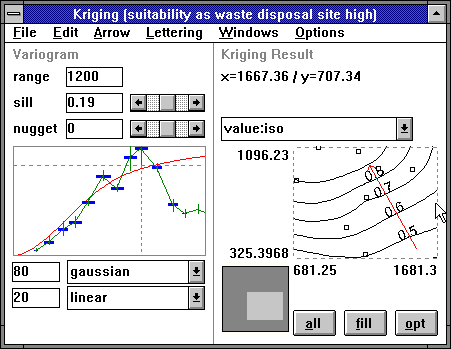FUZZEKS [to Index]
Handling Variograms

### The variogram display in FUZZEKS

In FUZZEKS both curves for experimental and theoretical variogram are displayed in the box on the left side of the kriging window:In the variogram window on the left side the distance is noted on the X-axis: In order to calculate the experimental variogram value for similar distances the X-axis is divided into sections. Each experimental variogram value is displayed as intersection of the vertical green lines and the horizontal blue lines. The length of the vertical green line indicates the fuzziness (this is defined in the corresponding options-menu by default, i.e. the top of the line indicates the largest possible value and the bottom indicates the lowest possible value).
The horizontal blue line indicates how many data points have been taken into account when calculating the experimental variogram value.

The red curve is the theoretical variogram (a function of the distance). It is determined by the input fields above and below the variogram window (user-supplied inputs, see Theoretical variogram fitting).

Both experimental and theoretical variogram use the same coordinate-system.

Note: If the range or sill value (of the theoretical variogram) are too small, the curves lie outside the display-area and no experimental variogram can be seen. If a new data set is used, one should use relatively large values for both fields first. The range can be overestimated by about the largest distance between two data points. The sill can be overestimated by about the half of the square of the largest difference between the values of two data points.

The next pages deal with